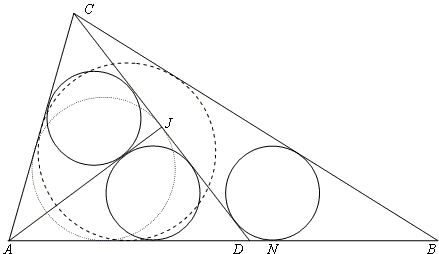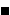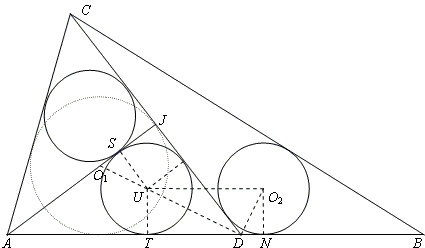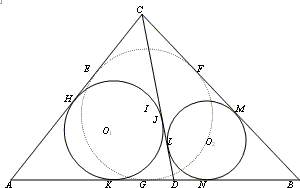## A better solution to a difficult sangaku problem

### J. Marshall Unger Department of East Asian Languages & Literatures The Ohio State University

PROBLEM. In ΔABC, AB = BC. If one chooses D on AB and J on CD such that AJ ⊥ CD and the incircles of ΔACJ, ΔADJ, and ΔBCD all have radius r, then r = AJ/4.SOLUTION

The trick to solving the problem expeditiously is to clarify what is given and to prove the converse proposition first.

Notice that the only connection between AB = BC and the geometry of ΔACD is that the incircle of ΔABC (dashes) is tangent to AC at its midpoint. The two incircles of ΔACJ and ΔADJ cannot be tangent to the shared side AJ at the same point, as shown, unless J is the point of tangency of the incircle of ΔACD (dots) with side CD [Honsberger, see also Three Incircles in a Triangle]. Hence AC = AD. And the two incircles cannot be congruent unless, in addition, either ∠CAJ = ∠DAJ or AJ ⊥ CD, in which case, ΔACJ = ΔADJ and the other condition follows. Therefore, given a fixed isosceles ABC, if the congruence of all three small incircles implies r = AJ/4, as the problem asserts, then the converse must also be true. Indeed, it is easier to prove.

In the figure above, let a, b, d, and h be the lengths of CD, AD = AC, DN, and AJ, respectively. Let r1 and r2 be the radii of the incircles of ΔACD and ΔBCD, respectively. We use two lemmas, which we will prove later:

 1 a - b = 2d 2 2 r1r2 = ad

Given h = 4r, square the equation for the inradius of a right triangle: b = h + a/2 - 2r = 2r + a/2 to get b² = 4r² + 2ar + a²/4, and equate this with the Pythagorean result b² = (a/2)² + h² = a²/4 + 16r². This yields a = 6r, which, with h = 4r, implies b = 5r. Hence, by Lemma 1, 2d = r.

Now area( ΔACD) = ha/2 = 12r² = r1s, where s is the semiperimter of ΔACD or 8r. Thus r1 = 3r/2 = 3d. Using Lemma 2, we find r2 = a/6 = r.From this, it is clear that solving the original problem comes down to proving, without knowing the value of h, that ΔACJ and ΔADJ are 3:4:5 right triangles.

It's easy to show that ΔDTU is similar to ΔDO2U. Hence DU is the mean proportional between DT and O2U = DT + DN. In terms of lengths, we therefore have

 a/2 - r + d = [r² + (a/2 - r)²] / (a/2 - r) (a/2 - r)² + d (a/2 - r) = r² + (a/2 - r)² d (a/2 - r) = r² ad/2 - dr = r² r1r - dr = r² r1 - d = r.

(Lemma 2 takes the form 2r1r = ad since we are assuming r2 = r.) Since JS = r, O1S = d. Thus JO1 = r + d = 3d = r1.

Hence, by Lemma 2, 2rr1 = 2(2d)(3d) = 12d² = ad, so a = 12d = 6r. Since a - b = 2d (Lemma 1), b = 10d = 5r. And because ADJ is a right triangle, a/2 = 3r and b = 5r imply h = 4r.The lemmas follow from the general case of any triangle ABC with incircle I. Draw a cevian CD and the incircles O1 and O2 of the resulting triangles ACD and BCD. Label the points of tangency of these incircles as shown. Then EH = GK = DN.PROOF: By equal tangents, CE = CF (1), and

 AE = AG BF = BG CM = CL DJ = DK AH = AK BM = BN CH = CJ DN = DL.

Subtracting the equations in second row from those in the first, we get

 EH = GK (2) FM = GN (3) CM - CH = JL (4) JL = DK - DN (5).

Equating the left and right sides of (4) and (5),

 CM + DN = DK + CH (CF + FM) + DN = DK + (CE + EH) (by 1) FM + DN = DK + EH (by 3) GN + DN = DK + EH (GN - DG) + DN = (DK - DG) + EH 2DN = GK + EH (by 2) DN = GK (6).

Linking equations (2) and (6), EH = GK = DN.Many more inferences can be drawn from this figure, but we need just two.Lemma 1 follows immediately if E and J are the midpoints of AC and CD, respectively. Then CD/2 - AC/2 = EH = DN. In the problem, as explained earlier, J must be the midpoint of CD. Requiring that ABC be isosceles forces E to be the midpoint of AC.

Lemma 2 in the general case is r1r2 = DJ·DL. The dashed lines in the figure show that

(r1 + r2)² + (DJ - DL)² = (O1O2)² = (r1 - r2)² + KN²,

but KN = DK + DN = DJ + DL by equal tangents. The rest is just algebra.

In introducing the solution of Kitagawa Moko (1764 -1833), Fukagawa and Rothman remark, "It is somewhat complicated and we hope that readers can find a simpler one." I do not know whether the solution presented above is what Fukagawa and Rothman had in mind, but Kitagawa's solution is certainly complicated. It proves Lemma 1 relying in part on the given that ΔABC is isosceles; finds expressions for r1, a, and d in terms of r and h; plugs these directly into Lemma 2; produces a third-degree equation in r and h through more painstaking algebra; rejects the roots h = ± r2 because they are inconsistent with the figure; and so concludes that h = 4r. In addition, Kitagawa's proof gives no hint that the lemmas hold even for scalene ABC, the true significance of requiring that ABC be isosceles, that the converse is true, or that 3:4:5 right triangles play a role.

### References

1. H. Fukagawa, A. Rothman, Sacred Mathematics: Japanese Temple Geometry, Princeton University Press, 2008
2. R. Honsberger, Episodes in nineteenth and twentieth-century Euclidean geometry, MAA, 1995## Sangaku